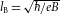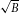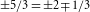## Main

The anomalous quantum Hall effect in graphene6,7, in which Hall plateaux appear at ν=±4(n+1/2) for Landau level index n=0,1,2…, can be understood using a theory of non-interacting, massless Dirac fermions in which the four-fold level degeneracy reflects the two spin and two valley degrees of freedom, and the 1/2 offset in the ladder of QHE states relates to the non-zero Berry phase acquired by Dirac fermions in a magnetic field. Each LL is approximately SU(4) symmetric, as symmetry breaking effects, such as Zeeman coupling and contact interactions, are weak at experimentally realizable magnetic fields. Even at B=35 T, the ratio between the Zeeman and Coulomb energies is EZ/EC0.01ε (where ε is the dielectric constant), and lattice scale interactions are only a/lB0.06 (where a is the lattice constant andis the magnetic length). Exchange interactions can favour ground states with an SU(4) ferromagnetic polarization9,10, yielding the observed18,19,20,21 IQHE at all integer ν in the cleanest samples. At fractional filling, the expanded symmetry of the LLs opens up the possibility of observing novel many-body states that have no analogue in one- and two-component systems12,14.

In this Letter we report on measurements of the FQHE in a single-layer graphene sample fabricated on a hexagonal boron nitride (h-BN) substrate (see Methods). Figure 1a shows the zero-field resistance and corresponding conductivity, acquired at T300 mK. The Hall mobility at high density is 30,000 cm2 Vs−1; the charged-impurity mobility, which dominates at low density, is in excess of 100,000 cm2 Vs−1, as determined by fitting straight lines to the linear portion of the conductivity; the charge inhomogeneity, estimated from the resistivity peak width at the charge neutrality point (CNP), is of the order 1010 cm−2. All three metrics indicate this sample to be of exceptionally high quality and are consistent with previous measurements of similar graphene/h-BN devices18.

Longitudinal (Rx x) and Hall (Rx y) magnetoresistance measurements acquired by varying the gate voltage at a fixed B field of 35 T are shown in Fig. 1c. Quantized Hall plateaux and magnetoresistance zeros appear at all accessible integer fillings in both the n=0 and n=1 LLs. Signatures of symmetry-breaking appear in the Shubnikov–de Haas (SdH) oscillations at fields as low as 1 T (inset of Fig. 1c), with full breaking of the four-fold degeneracy observed at fields less than 5 T. The most remarkable feature of this sample is the appearance of the FQHE at 35 T throughout the accessible density range (labelled with arrows in Fig. 1c). In the remainder of this Letter, we focus our attention on the FQHE states, with analysis of the integer QHE (IQHE) states to be given elsewhere.

In Fig. 2a,b, detailed plots of the n=0 and n=1 LLs are given. For clarity, the Hall conductivity, calculated from the tensor relation σx y=Rx y/(Rx y2+(w/l)Rx x2), is shown, where w/l is the aspect ratio of the Hall bar. We observe the hallmark features of the FQHE, namely quantization of Rx y to values of (1/ν)h/e2 concomitant with minima in Rx x, at fractional filling factors ν=1/3,2/3 and 4/3 in the n=0 LL and at ν=7/3,8/3,10/3,11/3 and 13/3 in the n=1 LL. Additionally, a weak minimum in Rx x at ν=8/5 is suggestive of an emerging FQHE state at this filling. With the exception of 1/3,2/3 and 7/3, all observed Hall conductivity plateaux are within 1% of their expected value. At 1/3 and 2/3, the Hall plateaux are ‘N’ shaped and do not exhibit exact quantization, typical of FQH states not yet fully formed in the magnetic field. The weak formation of the 1/3 and 2/3 states may be related to the presence of a competing, insulating phase at low density22 (see Supplementary Information).

The gap energies of the FQHE states were measured from the temperature dependence of the Rx x minima in the thermally activated regime; Rx x${e}^{\text{Δ}/2{k}_{\text{B}}T}$, where Δ is the energy gap, kB is Boltzmann’s constant and T is the electron temperature (Fig. 3). For all observed FQH states, the gaps are large in spite of considerable LL broadening due to disorder. For example, the measured gap at ν=4/3 is 16 K at 35 T. The large gaps probably result from the lower dielectric screening and near-zero width of the 2DES in comparison to conventional semiconductor heterostructures, both of which increase the effective strength of the electron interactions that give rise to the FQHE. Interestingly, the even-numerator and odd-numerator states exhibit opposite trends, with the even-numerator decreasing with increasing LL index, whereas the odd-numerator states actually grow in strength. Although the proximity of the 1/3 state to the insulating state does not allow us to measure Δ1/3, recent measurements performed on multiterminal suspended graphene indicate that Δ1/3 is indeed larger than any gap values measured in this device23. The trend in the gap scaling with filling fraction may be a consequence of the relativistic dispersion relation, which modifies the electron interactions in graphene11,24. In the context of the composite fermion theory, the fact that the even-numerator gaps are greater than those of the odd numerator is analogous to the broken symmetry IQHE of real electrons, where the even-integer states emerge from the otherwise four-fold degenerate LLs with stronger gaps than the odd-integer states19.

Measurement of the even-numerator gaps over a range of perpendicular fields is shown in Fig. 3d. In the simplest picture of spinless, non-interacting composite fermions, the FQHE energy gaps are set by the Coulomb interaction between real electrons and therefore exhibit a-dependence with magnetic field. However, the near degeneracy of the real spin can give rise to spin-textured charged excitations, resulting in a nearly linear dependence of the FQHE gaps on magnetic field25. A question of fundamental interest is whether incompressible states at fractional filling in graphene support spin-textured excitations, and how these relate to the underlying symmetry of the ground state. The solid and dashed lines in Fig. 3d are attempts to fit the data using square root and linear B -dependences, respectively. Both fit the data equally well, making it impossible to distinguish them at present. We use the y axis intercepts of the two fits to estimate the disorder-induced LL broadening to be in the range Γ10–30 K, giving an estimate for the intrinsic 4/3 gap at 35 T of 30–50 K, or 0.05–0.07 e2/ε lB in Coulomb energy units. Here we have taken the effective dielectric constant for graphene to be ε5, calculated from the internal electron screening due to interband transitions26, where the external dielectric environment is taken as an average of vacuum (εvac=1) and the underlying BN substrate (εBN3.5).

In the n=1 LL, the disorder limited 8/3 gap measures approximately 6 K. This is more than an order of magnitude larger gap than measured at the same total filling in GaAs devices27, where mobilities are in excess of 107 cm2 V−1 s−1, and remains 2–3 times larger even after accounting for the higher magnetic field at which our device was measured. This is consistent with the theoretical expectation that electron–electron interactions in the n=1 LL of graphene are expected to be similar in strength to the n=0 LL in GaAs (ref. 8).

No FQHE state appears in our samples at ν=5/3. As a result of the Berry phase shift in graphene, the lowest LL for electrons (holes) begins at filling factor ν=−2 (+2), in contrast to conventional zero-Berry phase materials, such as GaAs, in which the lowest LL begins at filling fraction ν=0. Filling factoris thus expected to be the closest analogue to the 1/3 state in conventional systems, making the non-observation of the 5/3 state conspicuous given that the 1/3 Laughlin state in conventional systems is generally robust. A distinguishing feature of graphene is the four-fold internal degeneracy, and in the presence of a large magnetic field we may consider three scenarios: (1) all degeneracies are lifted by coupling to external fields and FQHE states cannot mix spin/valley branches; (2) only one degeneracy, either spin or valley, is fully lifted, preserving an SU(2) symmetry in the remaining degenerate space and allowing, for example, mixed pseudospin but not mixed-spin FQH states; or (3) both Zeeman and valley splitting terms are sufficiently small that Coulomb interactions mix all branches, allowing mixed-spin/valley FQHE states and excitations. The remaining degeneracy should be reflected in the particle–hole symmetry of the FQHE states; in particular, we expect particle–hole conjugate FQH states within a single LL to have similar spin textures and gaps in the absence of any second order effects such as LL mixing28. The presence of the 1/3,2/3 and 4/3 together with the absence of the 5/3 is inconsistent with both scenarios (1) and (2), which require simultaneous observation of either 4/3 and 5/3, or 1/3 and 5/3, respectively (Fig. 2d). The absence of any particle–hole symmetry except across the full n=0 LL is therefore consistent with the scenario where symmetry breaking terms remain sufficiently small that an approximate SU(4) symmetry is preserved. We note that previously studied multicomponent 2DES such as AlAs and strained silicon28,29, in addition to differences in the orbital character of the wavefunctions, differ from graphene in that the larger ratio between Zeeman and Coulomb energies effectively freezes out the spin degree of freedom at large magnetic fields.

Whether an approximate SU(2) or SU(4) symmetry is assumed, a fully spin- and valley-polarized ground state with low-lying valley skyrmion excitations is theoretically expected at ν=5/3 (refs 8, 9, 11, 14, 15). Theoretical calculations for a corresponding valley skyrmion gap (0.03–0.043 e2/ε lB; refs 8, 16) are comparable to the 0.02–0.04 e2/ε lB LL broadening estimated from our measurement of the 4/3 gap, possibly explaining our non-observation of the 5/3 . At even-numerator filling in the lowest LL, such as at 8/5 and 4/3, a valley-unpolarized ground state is theoretically predicted8,11,14,16, with larger energy excitations than those occurring at 5/3, qualitatively consistent with our experimental findings. The smallness of the Zeeman energy in comparison with Coulomb energy theoretically allows for the possibility17 of real spin-reversed excitations at ν=1/3, and may account for the relative strength of the observed 1/3 in comparison with the absent 5/3. Alternatively, disorder may have a different effect near the edge of the LL (5/3) than it does near the insulating state at charge neutrality (1/3), possibly accounting for some of the observed difference. Further experimental and theoretical efforts in determining the real- and valley-spin texture of the FQHE ground states and their elementary excitations are necessary to resolve this puzzle.

The second LL (SLL) shows nearly all multiples of 1/3 within the experimental range. For example, the 7/3=2+(1/3) state, the SLL analogue of the 5/3, is present, and the 11/3=2+(5/3) and 10/3=2+(4/3), analogues of the 1/3 and 2/3, are also both well developed. Observation of the FQHE at ν=13/3 represents, to our knowledge, the first clear measurement of the FQHE at a filling factor ν>4. This may be attributed at least in part to the fact that the FQHE states between 4<ν<6 continue to belong to the n=1 LL because of the four-fold symmetry of single-particle LLs in graphene.

## Methods

To fabricate the graphene–on–h-BN device a similar mechanical transfer technique to that described in ref. 18 was used but with the water-soluble sacrificial layer replaced by a polyvinyl alcohol (PVA) layer. This allowed mechanical peeling of the PMMA membrane without the need to expose the graphene/PMMA substrate to a water bath, thereby achieving a fully dry transfer method. Electrical leads consisting of a Cr/Au metal stack were deposited using standard electron-beam lithography, after which the sample was etched into an approximately square Hall bar by exposure to oxygen plasma (Fig. 1b). Four-terminal transport measurements were performed using a lock-in amplifier at 17 Hz with a 10 nA source current. The sample was measured in a 35 T resistive magnet and 3He cryostat (sample in vapour). We note that there is an apparent asymmetry in the transport, with p-type carriers showing better developed quantum Hall effect features/higher mobility than n-type carriers, particularly in the second LL. This higher mobility for p-type carriers is not a systematic trend, as we have observed higher mobility for n-type carriers in other samples. Therefore, although data acquired at negative gate voltages (p-type carriers) is primarily discussed, we expect our results should be consistent with samples where n-type carriers experience comparable disorder.